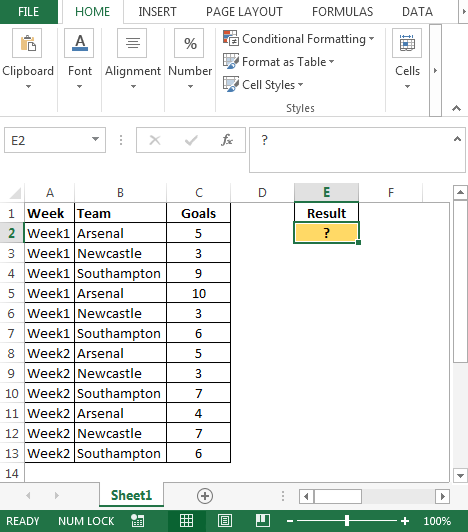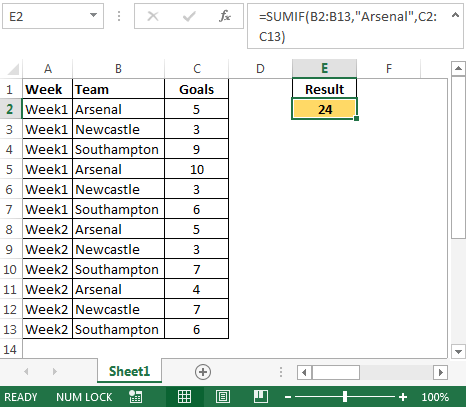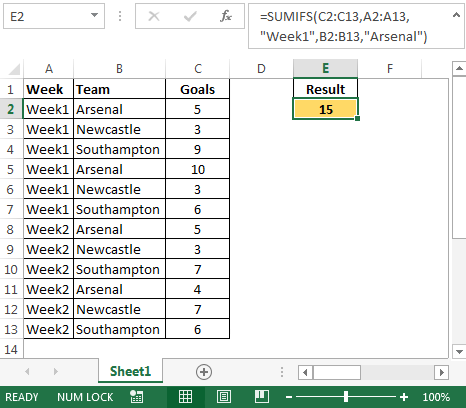How to Sum Matching Values From Multiple Rows in Microsoft Excel

To sum cells based on multiple criteria we can use SUMIF or SUMIFS function depending on the condition. If you want to learn SUMIFS function then this article is especially for you. In this article we will focus on how to sum values meeting matches values from multiple rows of data.

Question): I have a data comprising of Soccer Goals for each week; I want to calculate the goals by a particular team. Which formula I can use?

Following is the snapshot of data we have:• We can use SUMIF function to calculate the goals done by the specific team
• In cell E2 the formula will be
• =SUMIF(B2:B13,"Arsenal",C2:C13)To calculate a team’s highest goal in specific week we will use SUMIFS function.

• In cell E2 the formula will be
• =SUMIFS(C2:C13,A2:A13,"Week1",B2:B13,"Arsenal")In this way we can SUMIF & SUMIFS function to meet multiple conditions in various rows of data.1.